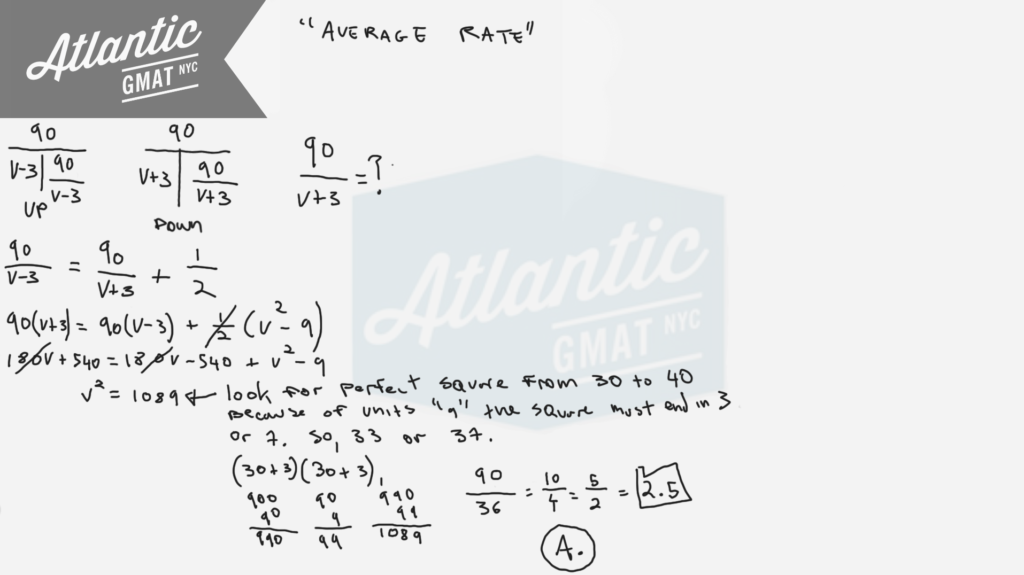Private GMAT Tutoring in NYC & Online

A boat traveled upstream a distance of 90 miles at an average speed of (v-3) miles per hour and then traveled the same distance downstream at an average speed of (v+3) miles per hour. If the trip upstream took a half hour longer than the trip downstream, then how many hours did it take the boat to travel downstream?

(A) 2.5
(B) 2.4
(C) 2.3
(D) 2.2
(E) 2.1

This is a GMAT Average Rate question from the GMAT Prep tests. If you haven't yet done the GMAT Prep Tests then better to avoid solving this now so you have a fresh test experience. I'd organize this using the T's. Just put the information where it belongs. Make sure to define the question: How many hours did it take the boat to travel downstream? Basically we need to solve for V and then plug that value into the downstream T to get the time. There are two challenging things about this question that I see troubling GMAT tutoring students:

1. Often the "half hour longer" gets translated into 30 minutes. That causes chaos. If you use minutes then everything must be changed to that unit. I wouldn't suggest taking that route here.
2. Quadratics freak people out and cause panic which leads to poor decision making. Just because there's a quadratic doesn't mean that it's going to take a lot of work to solve. Slow down. Think about it for a second. Try to make a plan. In this case we know that the square must be between 30 and 40 (30*30 = 900, 40*40 = 1600) and must create a units digit of 9 limiting our options to 33 and 37. You might say: "but how do we know it's going to be a perfect square"??? Well, do you see radicals in the answer choices??? Nope. Try 33 first because 1089 is much closer to 900 than 1600. Take your time with the arithmetic. I like to use distribution math for multiplication.Here are more Official GMAT distance, work, and rate question for which we use the T method: Pumps A, B, and C operate at their respective constant ratesHere's a bunch of extra practice questions focusing on average rates:

Distance, Work and Rate - Average Rate 1

Distance, Work and Rate - Average Rate 2

Distance, Work and Rate - Average Rate 3

For more GMAT distance, work, and rate practice visit GMAT Question of the Day.

# CONTACT

Atlantic GMAT

405 East 51st St.

NY, NY 10022

(347) 669-3545

info@AtlanticGMAT.com